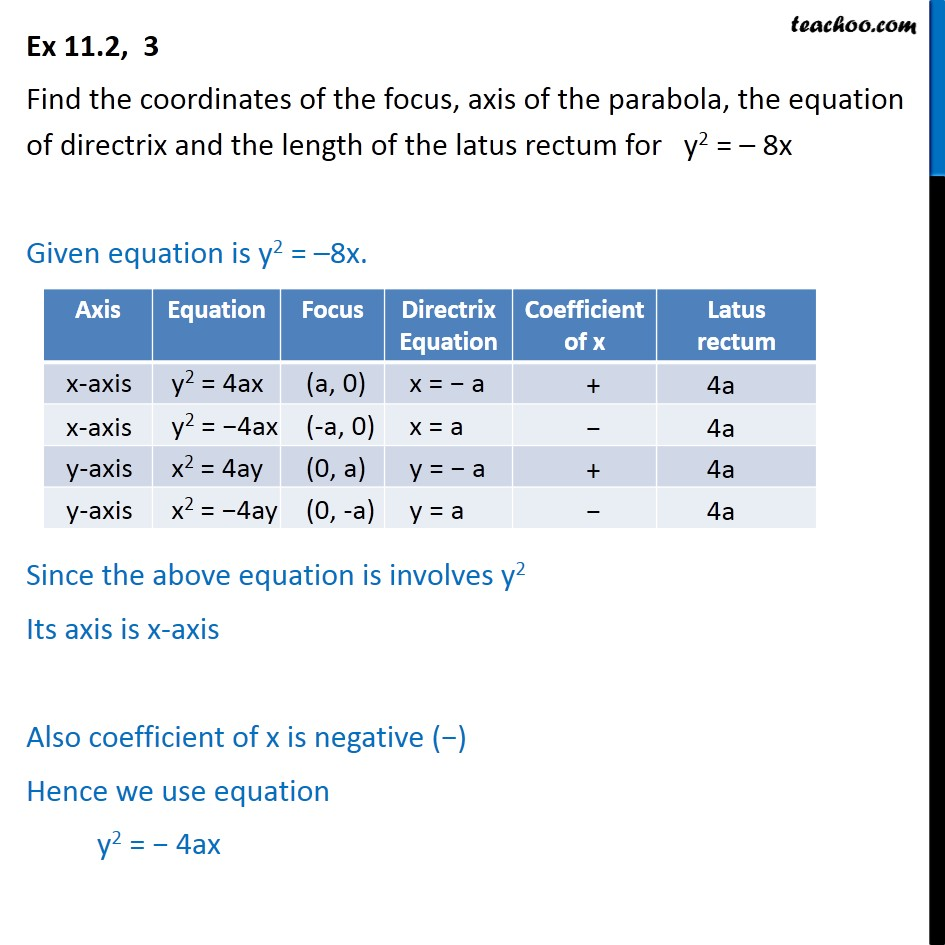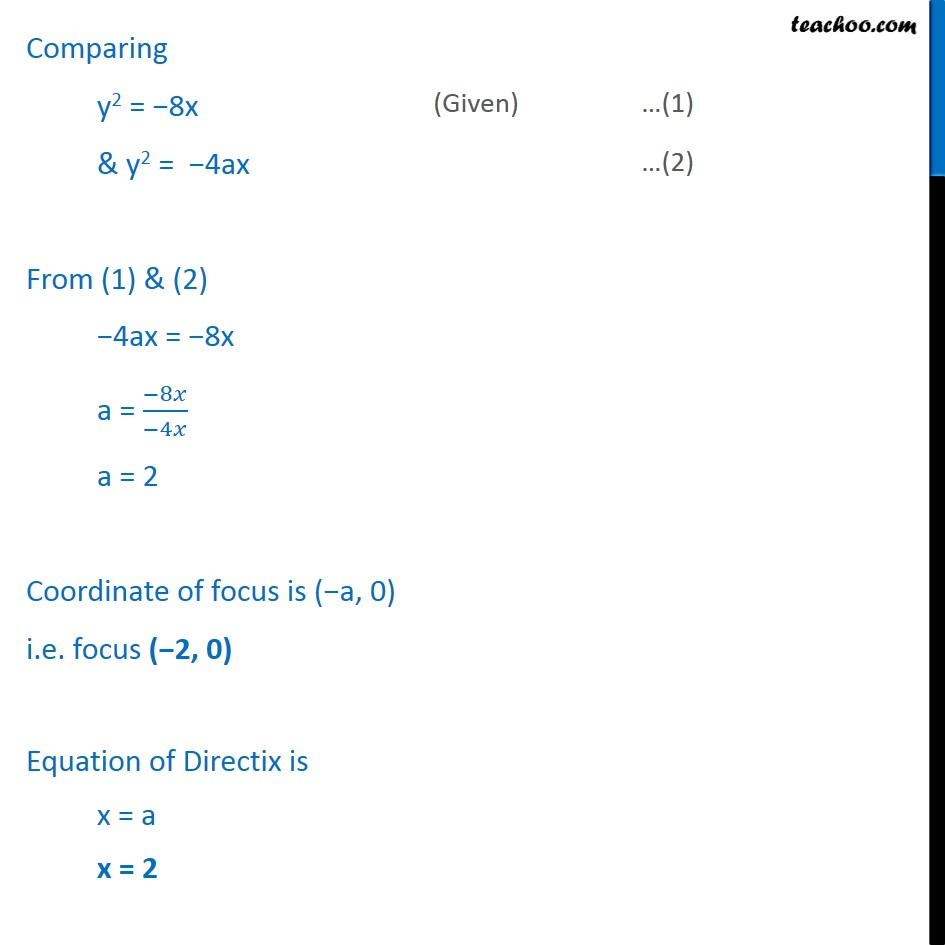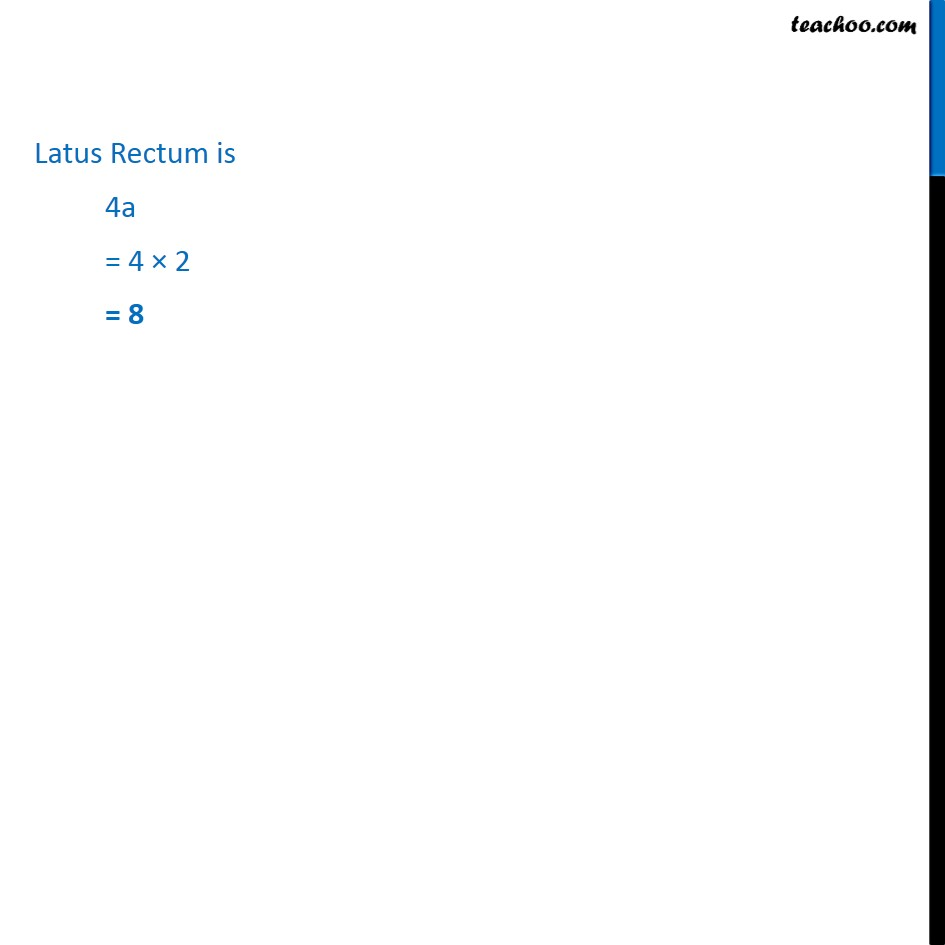1. Chapter 11 Class 11 Conic Sections
2. Serial order wise
3. Ex 11.2

Transcript

Ex 11.2, 3 Find the coordinates of the focus, axis of the parabola, the equation of directrix and the length of the latus rectum for y2 = 8x Given equation is y2 = 8x. Since the above equation is involves y2 Its axis is x-axis Also coefficient of x is negative ( ) Hence we use equation y2 = 4ax Latus Rectum is 4a = 4 2 = 8

Ex 11.2